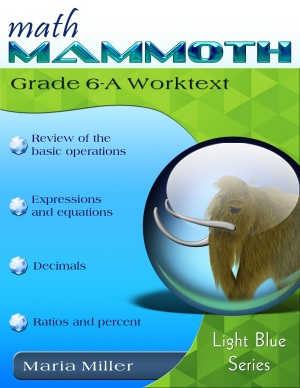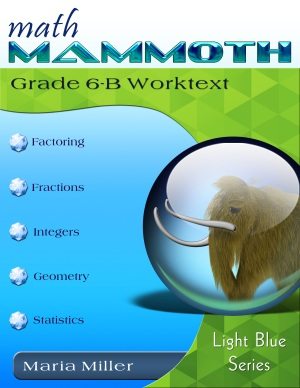^
You are here: HomeLight Blue Series → Grade 6

# Math Mammoth Grade 6 complete curriculum

Math Mammoth Grade 6 Complete Curriculum is a complete math program for 6th grade, providing you with everything you need to teach 6th grade math. It is available both as a download version and as printed copies.189 pages
170 lesson pages178 pages
153 lesson pages

## Prices & ordering

#### Digital version

(the two worktexts, answer keys, tests, cumulative reviews, a worksheet maker, Soft-Pak)

Part 6-A only:

(Everything for the first half of 6th grade math; includes Soft-Pak)

Part 6-B only:

(Everything for the second half of 6th grade math; includes Soft-Pak)

#### CD

(the two worktexts, answer keys, tests, cumulative reviews, a worksheet maker, Soft-Pak)

#### Printed copies

Rainbow Resource Center sells perfect-bound texts either with full color or with grayscale interior pages:

Lulu sells spiral (coil) bound versions of the student books and of the tests & reviews book.

6-A worktext \$17.45 (color)
6-B worktext \$16.95 (color)
Tests & Cumulative Reviews \$8.95 (color)

Another option is to purchase only the student worktexts as printed books, and get a digital version of the supportive materials: answer keys, tests, cumulative reviews, worksheet maker, and software Soft-Pak. Use this link to add the supportive materials to your shopping cart (\$8.95 USD).

#### Lesson plan

You can purchase a lesson plan for Math Mammoth Grade 6 to use it in Homeschool Planet online planner. Learn more.

At the heart of the curriculum are two student worktexts (A and B), each covering about half a year of math work. These worktexts contain all the instruction and the exercises in the same book — which makes lesson preparation a breeze. The worktexts are written directly to the student, and thus they allow many students to teach themselves and to learn directly from the books.

You will also get separate answer keys, chapter tests, additional cumulative reviews (I do recommend you use at least some of them), and a versatile worksheet maker (Internet access required) for those times when your child needs just a bit more practice.

## Features

• Math Mammoth focuses on conceptual understanding. It explains the "WHY", so your children can understand the math, not just learn "HOW" to do it.
• Concepts are often explained with visual models, followed by exercises using those models. These visual models can take the place of manipulatives for many children; however, it is very easy to add corresponding manipulatives to the lessons if so desired.
• The curriculum is mastery-oriented. This means it concentrates fairly long on a topic, delving into its various aspects. This promotes conceptual understanding, as opposed to spiral curricula that often tend to jump from topic to topic too much.
• Very little teacher preparation is required.• The curriculum has no separate teacher's manual nor is it scripted. The introduction to each chapter has some notes for the teacher concerning the material in the chapter. All the instruction is written directly to the student in the worktext, and there also exist accompanying videos where you can see Maria herself teach the material.
• After each chapter introduction, you will find a list of Internet links and resources (games, quizzes, animations, etc.) that can be used for fun, illustrations, and further practice.

The PDF files are enabled for annotation. This means that if you prefer, your student can fill them in on the computer, using the typewriter and drawing tools in Acrobat Reader version 9 or greater, or on a tablet device using any PDF app with annotation capabilities. (Learn more.)

BONUS! If you purchase the download or CD version, you will also get Soft-Pak programs completely FREE. The 6 programs in Soft-Pak (4 math, 1 language arts, 1 list maker) offer both on-screen and printable activities in a low-graphic, high content format. Read more and see screenshots.

## Overview of topics

The main areas of study in Math Mammoth Grade 6 are:

• review of the basic operations with whole numbers
• beginning algebra topics: expressions, equations, and inequalities
• review of all decimal arithmetic
• introduction to ratios and percent
• prime factorization, GCF, and LCM
• a review of fraction arithmetic from 5th grade, plus a focus on division of fractions
• the concept of integers, coordinate grid, addition & subtraction of integers
• geometry: review of quadrilaterals & drawing problems; area of triangles & polygons; volume of rectangular prisms with fractional edge lengths; surface area
• statistics: concept of distribution, measures of center, measures of variation, boxplots, stem-and-leaf plots, histograms

Please also see the table of contents 6-A and 6-B (in the sample files), which will let you see the topics covered in more detail.

## Supplements

### Lesson plan

We offer a lesson plan for Math Mammoth Grade 6 for the Homeschool Planet online planner. This is available in two versions: regular, and PLUS plan which also includes a PDF file of the plan. Learn more.

### Skills Review Workbook

Math Mammoth Skills Review Workbook, Grade 6 provides additional practice for the topics in Math Mammoth Grade 6 curriculum. I recommend you only purchase it once you are sure your student does need supplemental practice. Learn more.

## Introduction

In sixth grade, students encounter the beginnings of algebra, learning about algebraic expressions, one-variable equations and inequalities, integers, and ratios. We also review and deepen the students' understanding of rational numbers: both fractions and decimals are studied in depth, while percent is a new topic for 6th grade. In geometry, students learn to compute the area of various polygons, and also calculate volume and surface area of various solids. The last major area of study is statistics, where students learn to summarize and describe distributions using both measures of center and variability.

The year starts out with a review of the four operations with whole numbers (including long division), place value, and rounding. Students are also introduced to exponents and do some problem solving.

Chapter 2 starts the study of algebra topics, delving first into expressions and equations. Students practice writing expressions in many different ways, and use properties of operations and the idea of maintaining the equality of both sides of an equation to solve simple one-step equations. We also study briefly inequalities and using two variables.

Chapter 3 has to do with decimals. This is a long chapter, as we review all of decimal arithmetic, just using more decimal digits than in 5th grade. Students also convert measuring units in this chapter.

Ratios is a new topic (chapter 4). Students are already familiar with finding fractional parts from earlier grades, and now it is time to advance that knowledge into the study of ratios, which arise naturally from dividing a quantity into many equal parts. We study such topics as rates, unit rates, equivalent ratios, and problem solving using bar models.

Percent (chapter 5) is an important topic to understand thoroughly, because of its many applications in real life. The goal of this chapter is to develop a basic understanding of percent, to see percentages as decimals, and to learn to calculate discounts.

In chapter 6, we first review prime factorization, and then study the greatest common factor and the least common multiple.

Chapter 7 first presents a thorough review of fraction operations from 5th grade (addition, subtraction, and multiplication). The topic of focus in the chapter is fraction division. Students also do some problem solving with fractions.

In chapter 8, students are introduced to integers. They plot points in all four quadrants of the coordinate plane and learn to add and subtract with negative numbers. Multiplication and division of integers are studied in 7th grade.

In geometry (chapter 9), the focus is on the area of polygons. This is studied in a logical progression: first, the area of right triangles, then the area of parallelograms, then, the area of triangles, and lastly the area of polygons. We also review some topics from earlier grades (quadrilaterals, perimeter, basic drawing). Other major topics in this chapter are calculating surface area of some solids using nets, and volume of rectangular prisms with fractional edge lengths.

Lastly, in chapter 10, students study statistics. The focus idea is that of a statistical distribution. Students learn about measures of center and measures of variability. They learn how to make dot plots, histograms, boxplots, and stem-and-leaf plots. All of these lessons are necessary to summarize and analyze distributions.

## Reviews / Testimonials of the 6th grade curriculum

Taking a look at Math Mammoth — a review of MM6 by Jaime Gravitt at My Homeschool Story blog

Dear Maria,
You are awesome. Thanks so much for your amazing curriculum. I am using 6th and 7th grade books for my 7th grade daughter who is homeschooling this year. I love that your curriculum corresponds to state standards so when my daughter goes back to school she not be behind. My daughter has gone from a student who did not like math to one who does math first thing in the morning! Thanks again!!

October 2015

## How and where to order

You can buy Math Mammoth books at:

• Here at MathMammoth.com website — simply use the "Add to cart" buttons you see on the product pages.
• Rainbow Resource carries printed copies for the Light Blue series books, plus several CDs (Light Blue and Blue series).
• At Teachers Pay Teachers you can purchase the Light Blue Series downloads, plus topical units.
• Lulu sells printed copies for most of the Math Mammoth materials (various series).

By purchasing any of the books, permission IS granted for the teacher (or parent) to reproduce this material to be used with his/her students in a teaching situation; not for commercial resale. However, you are not permitted to share the material with another teacher.

In other words, you are permitted to make copies for the students/children you are teaching, but not for other teachers' usage.

Math Mammoth books are PDF files. You will need Adobe Reader to view them, including if you use a Mac or Linux. You can try other PDF viewers, but they seem to either omit or mess up some of the images.
WAIT!

Receive my monthly collection of math tips & resources directly in your inbox — and get a FREE Math Mammoth book!You can unsubscribe at any time.

### Math Mammoth Tour

Confused about the different options? Take a virtual email tour around Math Mammoth! You'll receive:

An initial email to download your GIFT of over 400 free worksheets and sample pages from my books. Six other "TOURSTOP" emails that explain the important things and commonly asked questions concerning Math Mammoth curriculum. (Find out the differences between all these different-colored series!)

This way, you'll have time to digest the information over one or two weeks, plus an opportunity to ask me personally about the curriculum.
A monthly collection of math teaching tips & Math Mammoth updates (unsubscribe any time)### "Mini" Math Teaching Course

This is a little "virtual" 2-week course, where you will receive emails on important topics on teaching math, including:

- How to help a student who is behind
- Troubles with word problems
- Teaching multiplication tables
- Why fractions are so difficult
- The value of mistakes
- Should you use timed tests
- And more!

A GIFT of over 400 free worksheets and sample pages from my books right in the very beginning.A monthly collection of math teaching tips & Math Mammoth updates (unsubscribe any time)
Enter your email to receive math teaching tips, resources, Math Mammoth news & sales, humor, and more! I tend to send out these tips about once monthly, near the beginning of the month, but occasionally you may hear from me twice per month (and sometimes less often).• A GIFT of over 400 free worksheets and sample pages from my books.